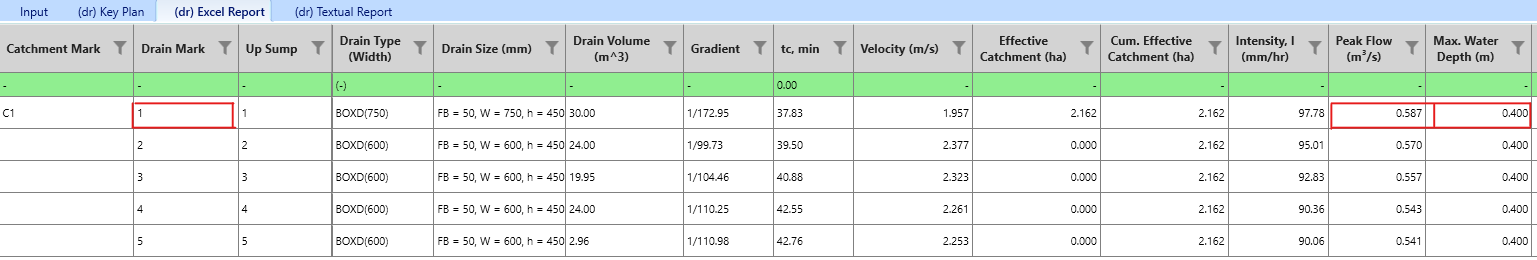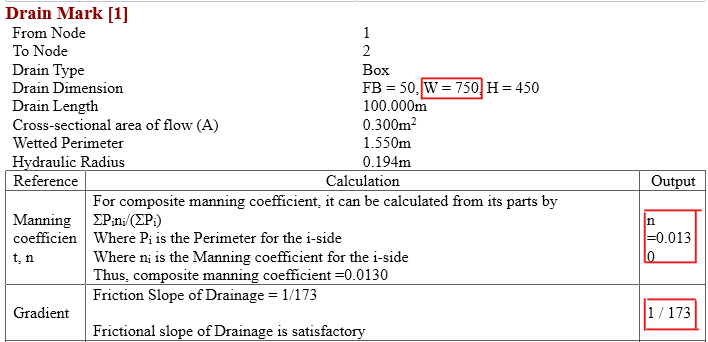603 - 5885 1250 (4 lines) info@mes100.com
Select Page

# How Does MiTS Calculate Maximum Water Depth in Drain?

Water depth is the height of the water surface as measured from the bottom of the water. Therefore, maximum water depth is the highest measurement from the bottom of the water. Maximum water depth occurs at the peak of the flow. So, how does MiTS calculate this maximum water depth? Given is an example of a box drain.

## Formula #

$$Q\:=\:vA$$

$$v\:=\:\frac{1}{n}\cdot R^{\frac{2}{3}}\cdot \sqrt{S}$$

where

$$R\:=\:\frac{A}{P}$$

Therefore,

$$Q\:=\:\left[\frac{1}{n}\cdot \left(\frac{A}{P}\right)^{\frac{2}{3}}\cdot \sqrt{S}\right]\cdot A$$

Q = peak flow, m3/s

n = manning coefficient

A = effective area (depth is maximum water depth in this case), m2

P = wetted perimeter (depth is maximum water depth in this case), m

S = drainage slope

## Box Drain, RC #

Project File

The example used was the Drain 1From the Excel & textual report,

Peak flow, Q = 0.587 m3/s

n = 0.013

Width = 0.75m

S = 1/173

Water depth = 0.4m, to calculate for the water depth, we placed it with y

Box Area, A = width x water depth

Bow wetted perimeter, P = water depth (2) + width

$$Q\:=\:\left[\frac{1}{n}\cdot \left(\frac{A}{P}\right)^{\frac{2}{3}}\cdot \sqrt{S}\right]\cdot A$$

$$0.587\:=\:\left[\frac{1}{0.013}\cdot \left(\frac{0.75y}{2y+0.75}\right)^{\frac{2}{3}}\cdot \sqrt{\frac{1}{173}}\right]\cdot 0.75y$$

$$0.007631\:=\:\frac{0.00975}{\sqrt{173}\cdot 0.013}\cdot \left(\frac{0.75y}{2y+0.75y}\right)^{\frac{2}{3}}\cdot y$$

$$4.4437E-7\:=\:\frac{5.21358E-7\cdot \:y^5}{\sqrt{173}\cdot 0.001520324y^2+\sqrt{173}\cdot 0.00140243y+\sqrt{173}\cdot 0.00021}$$

$$y\:\approx \:0.39996\:=\:4m$$

You can check the answer using this online calculator